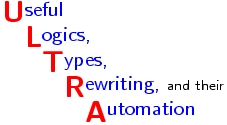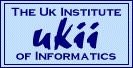# Abstracts of Workshops at School in Logic and Computation

## Heriot-Watt University, Edinburgh

### 10-13 April 1999ULTRA groupUseful Logics, Types, Rewriting, and ApplicationsUKII UK Institute of Informatics, UK

The list of abstracts is as follows:

Roberto Di Cosmo (Ecole normale superieure ENS, Paris), Invited Speaker

• Rewriting with extensionality
• Over the past ten years, much work has been done on typed lambda calculi with extensional axioms turned into expansive rules rather than into contractive ones. We will survey the rewriting techniques that have been developed and used for these calculi, which are a typical example of fruitful interaction between lambda calculus and rewriting, and the most recent results and applicatons in the field. Here are the slides.

Herman Geuvers (Eindhoven, NL) Invited Speaker :

• Inductive and Co-Inductive Types We will consider ways of defining types (and sets) by induction and coinduction. In doing so, we will avoid set-theory as much as possible, so our approach will be of a syntactical nature. The starting point will be second order predicate logic (PRED2); we will recollect the basic techniques of how to define inductive and coinductive predicates in PRED2. Based on that we will investigate further desires wrt induction and coinduction and compare existing approaches with ours. The basic questions to be answered will be
• Which inductive/coinductive types can we define?
• Which proof principles do we have on them?
• Which functions can we define on them?

Roy Dyckhoff (St Andrews, UK) invited speaker:

• Proof Search issues in constructive logic
• This talk will be an overview of my work (in part, jointly with Luis Pinto and sara Negri) on some intuitionistic sequent calculi, especially those with properties such as "contraction-free", "terminating" or "permutation-free" and which are organised for efficient proof search or solution of decision problems. In particular, we give proof-theoretic treatments of various completeness problems, such as that for a simple terminating contraction-free calculus, in which all the rules are invertible, for the G"odel-Dummett logic LC; and we discuss the termination of permutative reductions in sequent calculi and the relationship with Herbelin's work on his lambda-bar calculus.

Henk Barendregt Catholic University of Nijmegen and Sylvia Ghilezan University of Novi Sad,

• Lambda Terms for natural deduction, sequent calculus and cut elimination
• See full abstract

Roel de Vrijer, Free University of Amsterdam, Jan Willem Klop, Free University of Amsterdam, and Vincent van Oostrom, University of Utrecht,

• A GEOMETRIC PROOF OF CONFLUENCE BY DECREASING DIAGRAMS
• Recently a new confluence criterion for confluence was found using decreasing diagrams, as a generalization of several well-known confluence criteria in abstract rewriting such as the strong confluence lemma. We give a new proof of the decreasing diagram theorem based on a geometric study of infinite reduction diagrams, arising from unsuccesful attempts to obtain a confluent diagram by tiling with elementary diagrams.

Femke van Raamsdonk, Free University of Amsterdam,

• Higher-Order Rewriting
• In this talk I present a concise overview of higher-order term rewriting and I discuss some recent developments and trends.

Inge Bethke, Free University of Amsterdam, Jan Willem Klop, Free University of Amsterdam, and Roel de Vrijer, Free University of Amsterdam,

• EXTENDING PARTIAL COMBINATORY ALGEBRAS
• We give a negative answer to the question whether every partial combinatory algebra can be completed. The explicit counterexample will be an intricately constructed term model, the construction and the proof that it works heavily depending on syntactic techniques. In particular, it is a nice example of reasoning with elementary diagrams and descendants. We also include a domain-theoretic proof of the existence of an incompletable partial combinatory algebra.

Charles Stewart Brandeis University

• A Type Theory for Classical Arithmetic
• See abstract.ps

Samson Abramsky and Marina Lenisa Edinburgh University

• Realisability Models over linear combinatory algebras and the full completeness problem for typed lambda calculi
• See abstract.ps

Alexandru Baltag (CWI, Amsterdam)

• Truth as Simulation: Towards a Co-algebraic perspective on logic and games.
• Here is the the abstract

Didier Galmiche(Loria, Nancy):

• Some Issues about Proof Search in Linear Logic
• Here is the the abstract

• Iteration 2-categories and Rational Term Rewriting
• Here is the the abstract

Richard Kennaway, Vincent van Oostrom and Fer-Jan de Vries :

• Meaningless Terms in Rewriting
• We present an axiomatic approach to the concept of meaninglessness in finite and transfinite term rewriting and lambda calculus. We justify our axioms in several ways. They can be intuitively justified from the viewpoint of rewriting as computation. They are shown to imply important properties of meaninglessness: genericity of the class of meaningless terms, confluence modulo equality of meaningless terms, the consistency of equating all meaningless terms, and the construction of B\366hm trees and models of rewrite systems. Finally, we show that they can be easily verified for many existing notions of meaninglessness and easily refuted for some notions that are known not to be good characterizations of meaninglessness. Furio Honsell, Marino Miculan, and Ivan Scagnetto
• Pi calculus in Co-inductive Type Theory,
• Here is the the abstract

Jordi Levy and Margus Veanes (Spain)

• Undecidability of second-order unification
• Here is the the abstract

Jordi Levi and Mateu Villaret (Spain)

• Decidability of context unification
• Here is the the abstract

Randy Pollack University of Edinburgh and Katholic University of Nijmegen

• Names, Binding and Substitution
• If we take lambda-terms literally, problems of variable capture arise when defining substitution. Confusion between bound and free variables causes problems in other definitions as well, such as typing judgements. There are several well known approaches, e.g. nameless variables (de Bruijn indexes) and quotienting by alpha-conversion, but these have their own drawbacks. I will present an approach to binding and substitution using names that distinguishes between bound and free variables, and supports formal reasoning about terms as concrete objects (i.e. a datatype of terms). This approach has been used in a large formal development (by McKinna and Pollack) of some lambda calculus and type theory. I will compare this approach with some higher order representations recently proposed.

Hendrik Tews Universities of Nijmegen and Dresden

• Modeling binary methods: Some problems and results
• Coalgebras for endofunctors can be used to model classes of object oriented languages. Binary methods however do not fit directly into this approach. In this talk I will show how the original approach can be extended to solve this problem. I will introduce suitable notions of bisimulation and homomorphism and discuss some of the problems encountered.

Rene Vestergaard Heriot-Watt University

• Revisiting Kreisel: A Computational Anomaly in the Troelstra-Schwichtenberg G3i system
• Assuming a Curry-Howard Correspondence view on the Troelstra-Schwichtenberg G3i system, we will display a computational anomaly in its proof theory: it equates derivations (read: terms) that should be considered different. The problem comes about from the use of proof theoretical inversion in the intuitionistic setting. However, we will show that, in the end, it is caused by the use of over-zealous (explicit) contraction which stems from the system's lack of even a minimal notion of `assumption classes' (with associated implicit contraction). The initial analysis is carried out with a close eye to work on proof theory by Kreisel whose cautionary remarks we ultimately strengthen.

Wolfgang Windsteiger (Austria)

• Theorema: Overview on using the system
• Here is the the abstract